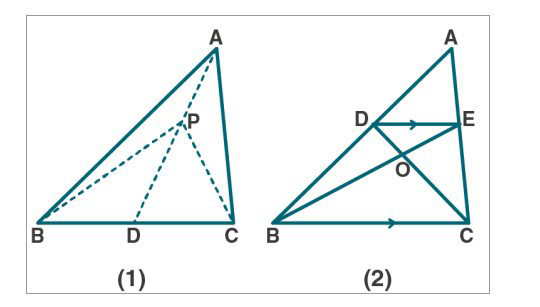# ML Aggarwal Solutions Class 9 Mathematics Solutions for Theorems on Area Exercise 14 in Chapter 14 - Theorems on Area

(a) In the figure (1) given below, AD is median of ∆ABC and P is any point on AD.

Prove that

(i) Area of ∆PBD = area of ∆PDC.

(ii) Area of ∆ABP = area of ∆ACP.

(b) In the figure (2) given below, DE || BC. Prove that

(i) area of ∆ACD = area of ∆ ABE.

(ii) Area of ∆OBD = area of ∆OCE.(a) Given:

∆ABC in which AD is the median. P is any point on AD. Join PB and PC.

To prove:

(i) Area of ∆PBD = area of ∆PDC.

(ii) Area of ∆ABP = area of ∆ACP.

Proof:

From fig (1)

AD is a median of ∆ABC

So, ar (∆ABD) = ar (∆ADC) …. (1)

Also, PD is the median of ∆BPD

Similarly, ar (∆PBD) = ar (∆PDC) …. (2)

Now, let us subtract (2) from (1), we get

ar (∆ABD) - ar (∆PBD) = ar (∆ADC) - ar (∆PDC)

Or ar (∆ABP) = ar (∆ACP)

Hence proved.

(b) Given:

∆ABC in which DE || BC

To prove:

(i) area of ∆ACD = area of ∆ ABE.

(ii) Area of ∆OBD = area of ∆OCE.

Proof:

From fig (2)

∆DEC and ∆BDE are on the same base DE and between the same || line DE and BE.

ar (∆DEC) = ar (∆BDE)

ar (∆ACD) = ar (∆ABE)

Hence proved.

Similarly, ar (∆DEC) = ar (∆BDE)

Subtract ar (∆DOE) from both sides, we get

ar (∆DEC) - ar (∆DOE) = ar (∆BDE) - ar (∆DOE)

ar (∆OBD) = ar (∆OCE)

Hence proved.

Related Questions
Exercises

Lido

Courses

Teachers

Book a Demo with us

Syllabus

Maths
CBSE
Maths
ICSE
Science
CBSE

Science
ICSE
English
CBSE
English
ICSE
Coding

Terms & Policies

Selina Question Bank

Maths
Physics
Biology

Allied Question Bank

Chemistry
Connect with us on social media!